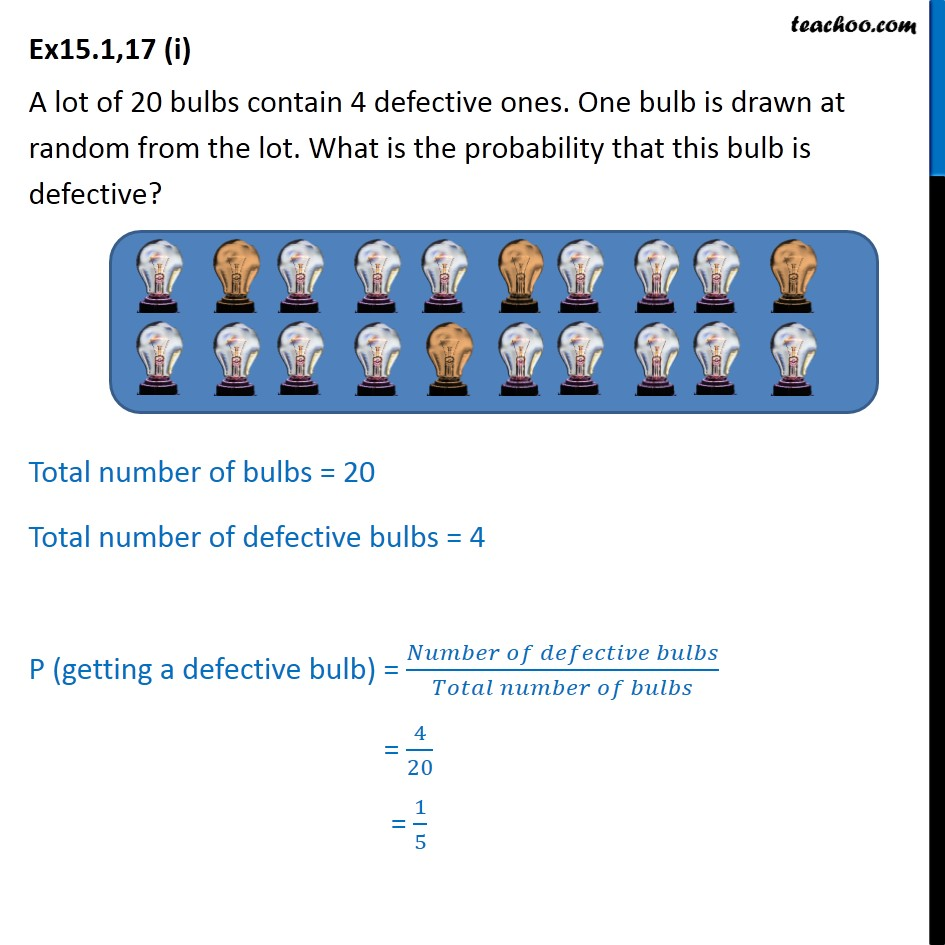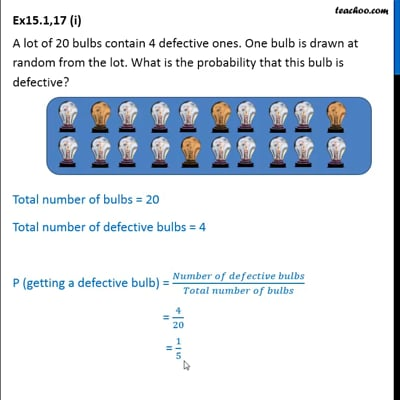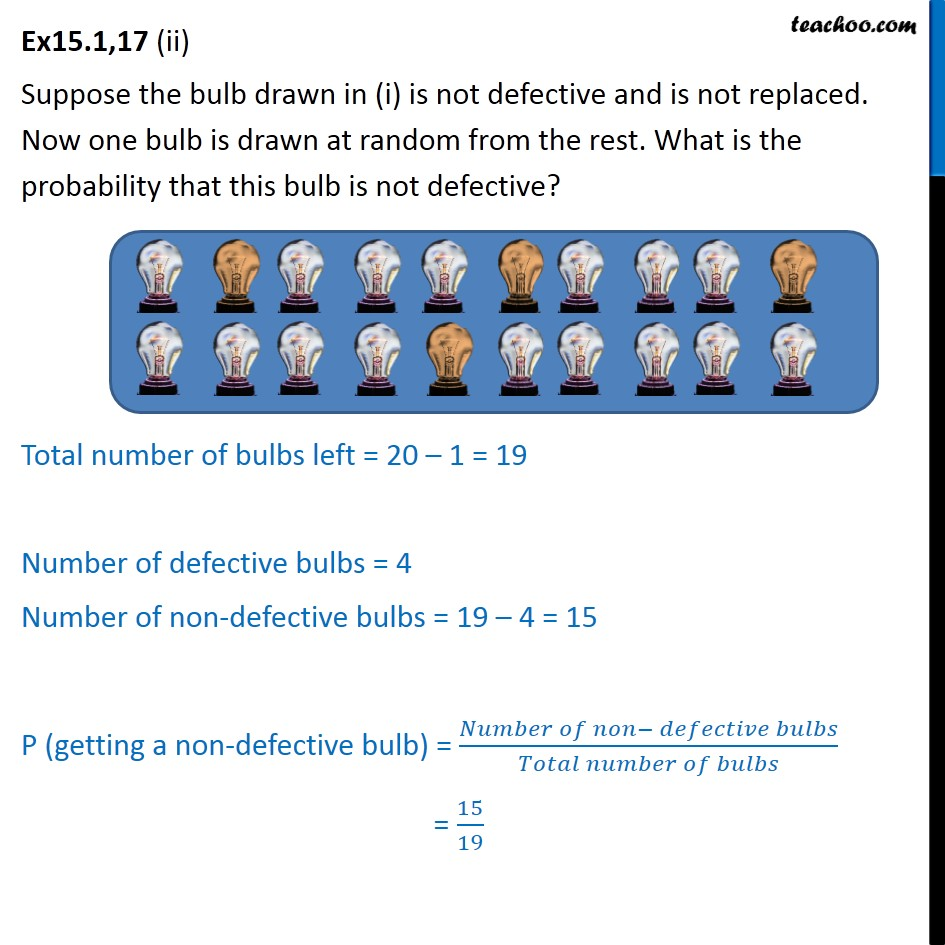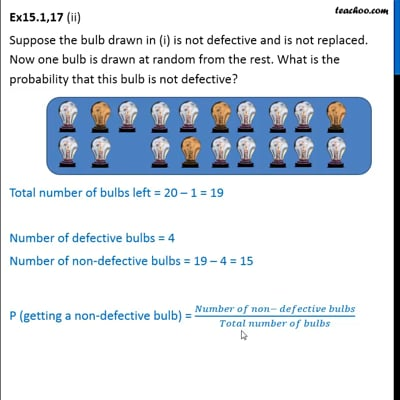Ex 15.1

Chapter 15 Class 10 Probability
Serial order wiseThis video is only available for Teachoo black usersThis video is only available for Teachoo black users

Maths Crash Course - Live lectures + all videos + Real time Doubt solving!

### Transcript

Ex15.1,17 (i) A lot of 20 bulbs contain 4 defective ones. One bulb is drawn at random from the lot. What is the probability that this bulb is defective? Total number of bulbs = 20 Total number of defective bulbs = 4 P (getting a defective bulb) = ( )/( ) = 4/20 = 1/5 Ex15.1,17 (ii) Suppose the bulb drawn in (i) is not defective and is not replaced. Now one bulb is drawn at random from the rest. What is the probability that this bulb is not defective? Total number of bulbs left = 20 1 = 19 Number of defective bulbs = 4 Number of non-defective bulbs = 19 4 = 15 P (getting a non-defective bulb) = ( )/( ) = 15/19